• 最近，S.MOshe和D.Hertz提出一个方法：用一个N维复向量的DFT计算一个N维实向量的DFT和另一个N维实向量的DFT的IDI。这是一个优美的结果，具有理论意义和应用价值。如何把上面的结果推广到二维的情形，是一个值得研究...
• 咱们只谈干货，二维DFT和退化模型的基础知识什么的就不在这说了，可以去看别的博主。 如果尝试去写的话遇到的问题可能有：①使用四个for循环的2维DFT运行时间比较长，我用实验室的i5试了下大概100分钟的样...
今天起床脑仁疼，趁着脑袋不清醒把这个月的工作总结一下haha，提前祝csdn和各位小老板双11快乐哈。

基于课业要求或者想了解matlab内库的童鞋想自己写DFT的和退化模型的可以看下。

咱们只谈干货，二维DFT和退化模型的基础知识什么的就不在这说了，可以去看别的博主。

如果尝试去写的话遇到的问题可能有：①使用四个for循环的2维DFT运行时间比较长，我用实验室的i5试了下大概100分钟的样子。考虑到matlab的老本行，还是用矩阵写。②矩阵的话"市面上"只有一维的，对于二维的其实不难猜到它的形式其实就是一维的推广。对一个MXN的矩阵进行运算得到一个MXN的可能会猜到就是MMXMNXNN，我写了两张A2的草稿纸证明了下是完全正确的。③退化函数Fspecial可能有人用的贼6，但是其内部机理还是有点说头的。好像如果只是按照给的H函数去做不会做出斜向的退化效果，这个时候就要湿考辽。

%%
%二维DFT
clc;
clear;
Q=imread('im.bmp');
I1=rgb2gray(Q);                                  %读取后转为灰度图
I=double(I1);
[M,N] = size(I);
%构造两个矩阵分别为MxM，NxN，在I前后分别相乘
m=[1:M];
n=[1:N]
subplot(221);imshow(I1);title('时域图像');
WM=exp(-j*2*pi/M);
mm=m'*m;
WMmm=WM.^mm;
WN=exp(-j*2*pi/N);
nn=n'*n;
WNnn=WN.^nn;                                     %是nn里的元素分别乘方，指数为WN
F=WMmm*I*WNnn;                                   %F里的元素均为复数
FRE=abs(F);                                    %取模并缩小范围
FRE=log(FRE+1);
%min=min(FRE(:));
%max=max(FRE(:));
FRE_=im2double(FRE);%转换为unit8便于显示
FRE_=FRE_/25;
y=fftshift(FRE_);
subplot(222);imshow(y);title('频域DFT图像');
%imwrite(y,'dft队员.bmp');

使用冈萨雷斯书里经典的配图，效果如下：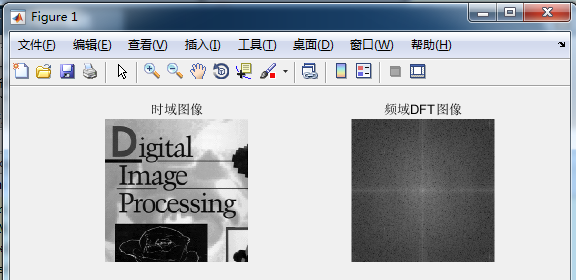可能有一些亮度上的缺陷，自行调整就好了。

clc,clear;
[I,map]=imread('im.bmp'); % 原始图像X数据类型为uint8
f=double(rgb2gray(I));
[M,N]=size(f);
% imshow(X,[]);title('ImageOrg.bmp');
H = zeros(M,N);
T=1;a=0.1;b=0.1;
for i = 1:M
for k = 1:N
ii=i-M/2+0.001;
kk=k-N/2+0.001;
H(i,k) = (T/(pi*(ii*a+kk*b))) * sin(pi*(ii*a+kk*b))*exp(-j*pi*(ii*a+kk*b));
end
end
F=fft2(f);
F=fftshift(F);
G=F.*H;
g=ifftshift(G);
gg=ifft2(g);
ggg=abs(gg)/200;
%gg=abs(g)/3;
imshow(ggg);title('result.bmp');

先解释下突破点，就是H函数其实原点在中心，而不是简单地把它带进去算，得到矩阵再相乘。然后有个小问题是会有ii=kk=0，这样分母就会为0，得到NAN，因此在后面加个很小的数（比如0.001）就行了。

退化没有用自己的DFT，变换部分直接用的库函数，效果如下：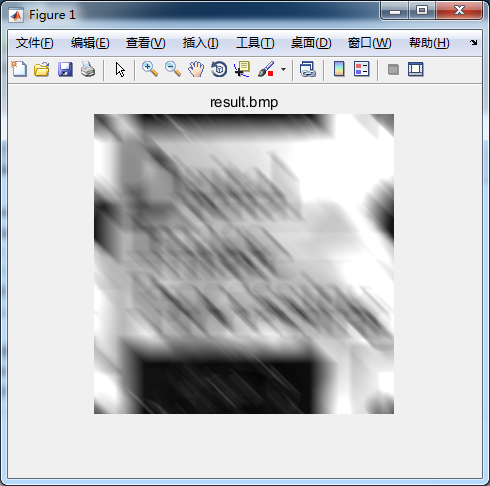用fspecial函数的效果如下，自己写的这个就算是成功了罢：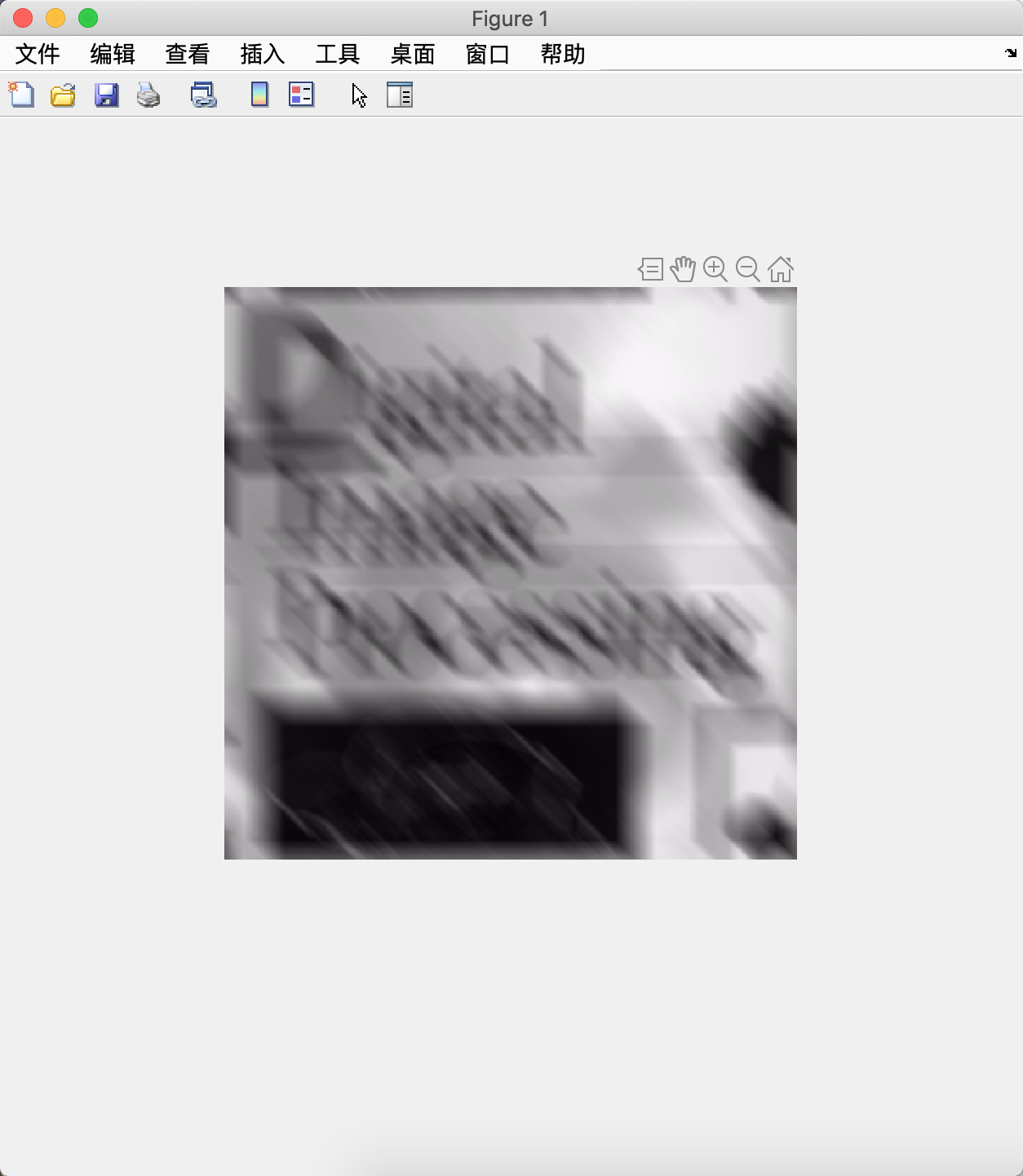展开全文• ## DFT和IDFT分析

千次阅读 2019-08-26 20:45:00
DFT和IDFT分析DFT和IDFT的意义DFT的定义DFT的矩阵分析 DFT和IDFT的意义 DFT：离散傅里叶变换 离散傅里叶变换可以将连续的频谱转化成离散的频谱去计算，这样就易于计算机编程实现傅里叶变换的计算。FFT算法的出现...
DFT和IDFT分析DFT和IDFT的意义定义DFT的定义DFT的定义DFT的矩阵分析DFT在matlab相关的代码分析
DFT和IDFT的意义
DFT：离散傅里叶变换
离散傅里叶变换可以将连续的频谱转化成离散的频谱去计算，这样就易于计算机编程实现傅里叶变换的计算。FFT算法的出现，使得DFT的计算速度更快。
定义
DFT的定义
设 $x(n)$ 是一个长度为$M$的有限长序列，则定义$x(n)$的$N$点离散傅里叶变换为
$X(k) = DFT[x(n)] = \sum\limits_{n = 0}^{N - 1} {x(n)W_N^{kn}} \ \ \ \ \ \ \ (k=0, 1, ..., N-1)$
注意：$x(n)$的离散傅里叶变换结果与变换区间长度$N$的取值有关。
DFT的定义
$X(k)$的离散傅里叶逆变换（Inverse Discrete Fourier Transform, IDFT）为
$x(n) = IDFT[X(k)]=\frac{1}{N} \sum\limits_{n = 0}^{N - 1} {X(k)W_N^{-kn}} \ \ \ \ \ \ \ (n=0, 1, ..., N-1)$
式中， $W_{N}=e^{-j\frac{2\pi}{N}}$, $N$称为DFT变换区间长度，$N \ge M$
DFT的矩阵分析
由DFT的数学表达式可以看出：

对于每一个$X(k)$的计算，为两个向量的内积形式

对于$k=0, 1, ..., N-1$,则$X(k)$的计算可以看做向量$x(n)$和一个矩阵的乘积，我们一般讲这个矩阵称作DFT矩阵。$X(k) = DFT[x(n)] = \sum\limits_{n = 0}^{N - 1} {x(n)W_N^{kn}} = x(n)*P \ \ \ \ \ \ \ (k=0, 1, ..., N-1)$        $P= \left[ \begin{array}{l} W_N^{0*0}\ \ W_N^{0*1} \ \ ...W_N^{0*n}\\ W_N^{1*0}\ \ W_N^{1*1} \ \ ...W_N^{1*n}\\ ... \\ W_N^{k*0}\ \ W_N^{k*1} \ \ ...W_N^{k*n}\\ \end{array} \right]$

同理，对于IDFT的运算，同样会有一个对应的IDFT矩阵，且和DFT矩阵有如下的关系: $x(n) = IDFT[X(k)]=\frac{1}{N} \sum\limits_{n = 0}^{N - 1} {X(k)W_N^{-kn}} =\frac{1}{N} X(k)*Q \\ (k=0, 1, ..., N-1)$        $Q= \left[ \begin{array}{l} W_N^{-0*0}\ \ W_N^{-0*1} \ \ ...W_N^{-0*n}\\ W_N^{-1*0}\ \ W_N^{-1*1} \ \ ...W_N^{-1*n}\\ ... \\ W_N^{-k*0}\ \ W_N^{-k*1} \ \ ...W_N^{-k*n}\\ \end{array} \right]$

对$P$和$Q$的形式进行分析，则可得到如下结论:
$Q = P^{*}$
其中，$P$是$DFT$矩阵，$Q$是$IDFT$矩阵，且$P$和$Q$均为对称阵。
$P$和$Q$的第一行和第一列全为1。

将$P$和$Q$应用于DFT和IDFT中，则有
$X(k) = DFT[x(n)] = x(n)*P \\ x(n) = IDFT[X(k)]=\frac{1}{N} X(k)*Q =\frac{1}{N} x(n)*P*Q \\ \frac{1}{N} x(n)*P*Q = I_{N}$
$P*Q = N * I_{N}$
说明$DFT$矩阵$P$和$IDFT$矩阵$Q$均为正交矩阵（$A*A^{H}=E$）。
这两个矩阵的每一行（列）都是有个基，在N个方向上都有不同的基向量。
因此，$DFT$和$IDFT$都是一种正交变换。

DFT在matlab
对于matlab中的$DFT$正变换，可使用内置函数dftmtx(n)，而对于$IDFT$的矩阵，可以通过$\frac{1}{N}*dftmtx(N)^{*}$来得到。
相关的代码分析
%% Prepare
clc;
close all;
clear;

%% Define the signal
N = 8;
x = [0:N-1];

%% Generate the vector of n and k
N = length(x);
n = [0:N-1];
k = [0:N-1];

%% Generate the twist-factor W
imag_unit = sqrt(-1);
W = exp(-1*imag_unit*2*pi/N);

%% Generate P matrix
P = W.^(n'*k);

%% DFT transformation
X_P = x*P;
X_fft = fft(x);
X_err = norm(X_P - X_fft)

%% Generate Q matrix
Q = conj(P);

% IDFT transformation
x_Q = 1/N * X_P * Q;
x_ifft = ifft(X_fft);
x_err = norm(x_Q - x_ifft)

%% Test: P*Q == N*I_n
I_n = eye(N);
left = P*Q;
right = N*I_n;
err = norm(left - right)

%% Generate P and Q using dftmtx
P_dftmtx = dftmtx(N);
P_err = norm(P_dftmtx - P)

Q_dftmtx = conj(dftmtx(N));
Q_err = norm(Q_dftmtx - Q)




展开全文FFT
• 这种 DFT 在变换的向量矩阵表示中是直观的，并且在内存时间之间的交易中是有效的（避免正弦、余弦计算循环）。 规范化也很简单。matlab
• 输入是大小为15X15的2D矩阵DFT / DFT.py文件具有函数“ forward_transform”，“ inverse_transform”，“ discrete_cosine_tranform”“ magnitude” 我已经为所有计算实现了自己的代码，没有使用numpy，...
• DFT的matlab源代码工作流程说明 运行计算： python3 tmd/bilayer/dgrid.py ：为d的网格（层间偏移）准备输入，并提交所有d的DFT计算（scf，nscf波段，nscf）。 python3 tmd/bilayer/submit_pw_...依赖项安装：LS5 安
• DFT的matlab源代码VASP中的职业矩阵控制 职业矩阵控件设置在计算DFT + U校正时使用的职业。 它不会直接改变电子分布。 这样，它将有效地鼓励进入的职业。 这有两个直接用途 设置特定的电子职业。 要获得特定位置...
• 在图像处理领域，通过DFT可以将图像转换到频域，实现高通低通滤波；还可以利用矩阵的卷积运算等同于其在频域的乘法运算从而优化算法降低运算量， 即先将图像转换到频域，然后做完乘法运算后，再转换到图像域，...

这次介绍下OpenCV中DFT的使用，对应的例程是(EXAMPLE) dft。在图像处理领域，通过DFT可以将图像转换到频域，实现高通和低通滤波；还可以利用矩阵的卷积运算等同于其在频域的乘法运算从而优化算法降低运算量，
即先将图像转换到频域，然后做完乘法运算后，再转换到图像域，opencv中的模板匹配就利用了这一特性降低运算量。

下面是dft例程的源码

[cpp] view
plain copy#include "opencv2/core/core.hpp"
#include "opencv2/imgproc/imgproc.hpp"
#include "opencv2/highgui/highgui.hpp"

#include <stdio.h>

using namespace cv;
using namespace std;

static void help()
{
printf("\nThis program demonstrated the use of the discrete Fourier transform (dft)\n"
"The dft of an image is taken and it's power spectrum is displayed.\n"
"Usage:\n"
"./dft [image_name -- default lena.jpg]\n");
}

const char* keys =
{
"{1| |lena.jpg|input image file}"
};

int main(int argc, const char ** argv)
{
help();
CommandLineParser parser(argc, argv, keys);        // opencv中用来处理命令行参数的类
string filename = parser.get<string>("1");

Mat img = imread(filename.c_str(), CV_LOAD_IMAGE_GRAYSCALE);    //以灰度图像读入
if( img.empty() )
{
help();
printf("Cannot read image file: %s\n", filename.c_str());
return -1;
}
int M = getOptimalDFTSize( img.rows );                               // 获得最佳DFT尺寸，为2的次方
int N = getOptimalDFTSize( img.cols );                                 //同上
Mat padded;
copyMakeBorder(img, padded, 0, M - img.rows, 0, N - img.cols, BORDER_CONSTANT, Scalar::all(0));   // opencv中的边界扩展函数，提供多种方式扩展

Mat planes[] = {Mat_<float>(padded), Mat::zeros(padded.size(), CV_32F)};          // Mat 数组，第一个为扩展后的图像，一个为空图像，
Mat complexImg;
merge(planes, 2, complexImg);                                                                              // 合并成一个Mat

dft(complexImg, complexImg);                                                                              // FFT变换， dft需要一个2通道的Mat

// compute log(1 + sqrt(Re(DFT(img))**2 + Im(DFT(img))**2))
split(complexImg, planes);                                                                                     //分离通道， planes 为实数部分，planes为虚数部分
magnitude(planes, planes, planes);                                                          // 求模
Mat mag = planes;
mag += Scalar::all(1);
log(mag, mag);                                                                                                      // 模的对数

// crop the spectrum, if it has an odd number of rows or columns
mag = mag(Rect(0, 0, mag.cols & -2, mag.rows & -2));                                        //保证偶数的边长

int cx = mag.cols/2;
int cy = mag.rows/2;

// rearrange the quadrants of Fourier image                                                        //对傅立叶变换的图像进行重排，4个区块，从左到右，从上到下 分别为q0, q1, q2, q3
// so that the origin is at the image center                                                          //  对调q0和q3, q1和q2
Mat tmp;
Mat q0(mag, Rect(0, 0, cx, cy));
Mat q1(mag, Rect(cx, 0, cx, cy));
Mat q2(mag, Rect(0, cy, cx, cy));
Mat q3(mag, Rect(cx, cy, cx, cy));

q0.copyTo(tmp);
q3.copyTo(q0);
tmp.copyTo(q3);

q1.copyTo(tmp);
q2.copyTo(q1);
tmp.copyTo(q2);

normalize(mag, mag, 0, 1, CV_MINMAX);                                                           // 规范化值到 0~1 显示图片的需要

imshow("spectrum magnitude", mag);
waitKey();
return 0;
}
下图为运行结果，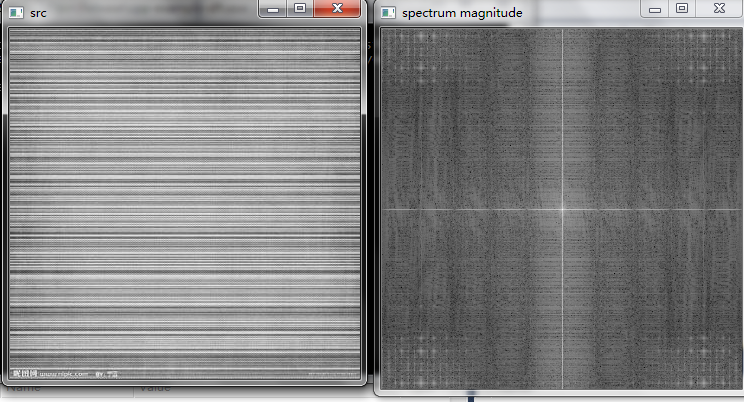例程只介绍了将图像转换到频域，那如何将其逆变换转换成图像呢？opencv中提供逆变换的函数为idft，下面看下dft，idft这两个函数的原型

[cpp] view
plain copy//! performs forward or inverse 1D or 2D Discrete Fourier Transformation
CV_EXPORTS_W void dft(InputArray src, OutputArray dst, int flags=0, int nonzeroRows=0);
//! performs inverse 1D or 2D Discrete Fourier Transformation
CV_EXPORTS_W void idft(InputArray src, OutputArray dst, int flags=0, int nonzeroRows=0);
从描述可以看出，dft，idft分别用于实现1维，2维的傅立叶变换和傅立叶逆变换。此时你或许可能认为opencv为dft，idft分别写了两个函数实现，但如果查看idft的源码，你会发现idft的实现也是在dft函数中，opencv中代码的重用率还是挺高的。

[cpp] view
plain copyvoid cv::idft( InputArray src, OutputArray dst, int flags, int nonzero_rows )
{
dft( src, dst, flags | DFT_INVERSE, nonzero_rows );
}
通过一个flags来控制是进行dft变换还是idft变换，这种用法在opencv中十分的普遍。我们可以在程序中添加一些代码

[cpp] view
plain copyMat ifft;
idft(complexImg,ifft,DFT_REAL_OUTPUT);
normalize(ifft,ifft,0,1,CV_MINMAX);
imshow("idft",ifft);
实现DFT的逆变换

结果如下图所示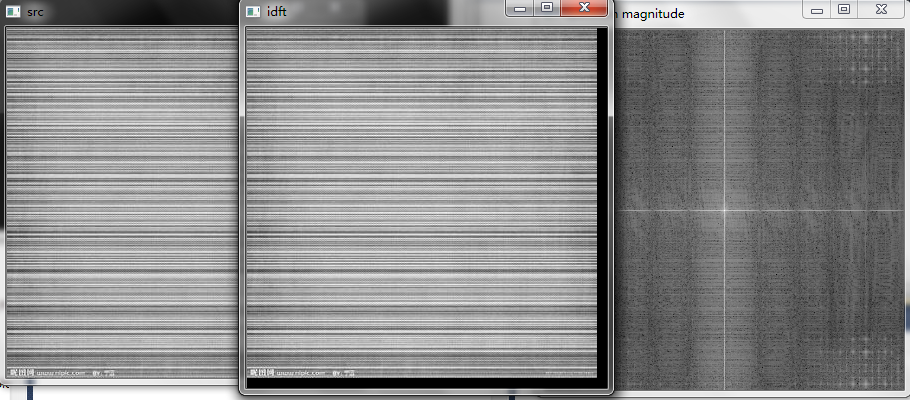可以看出逆变换后的图像内容与原图是一样的，因为我们进行任何处理，但是idf的大小比原图要大一些，且有些黑色边界，这是由于dft需要保证边长长度为2的次方，在这种情况下，计算速度可以加速，因此在之前的处理过程中，将原图的大小调整到2的次方，黑色边界便是copyMakeBoder的0值填充所形成的，这个函数在插值，滤波，掩模操作中十分常用，被用来扩展边界以是图像边界上的像素也能得以处理，只不过在那些操作中，处理完的图片与原图一样大小。

傅立叶变换还有一个特性经常被利用到，就是它的相位，用来确定图像的选择角度，例如tutorial文档中就提到一个应用，用来确定文字的旋转角度，我们可以通过将一张图像旋转一定的角度来做一个实验，下面是结果。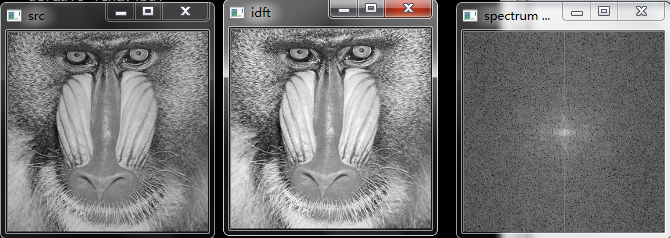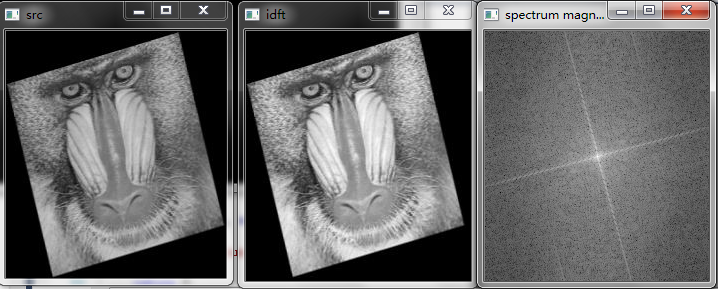可以看出沿着中心的两个轴随着图像的旋转而旋转，因此通过确定轴的旋转角度，便可以将图像位置调整过来，相应的，处理的如果是文字或者是车牌，也可以通过类似的处理进行对齐和角度调整。

对频域图像阈值处理后，可以看得更加清楚。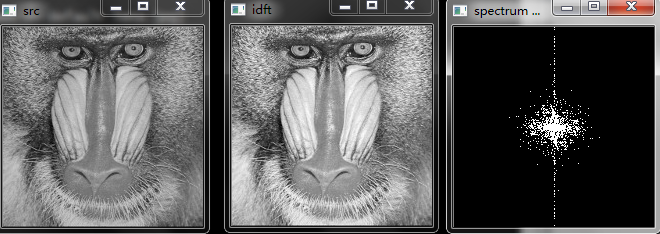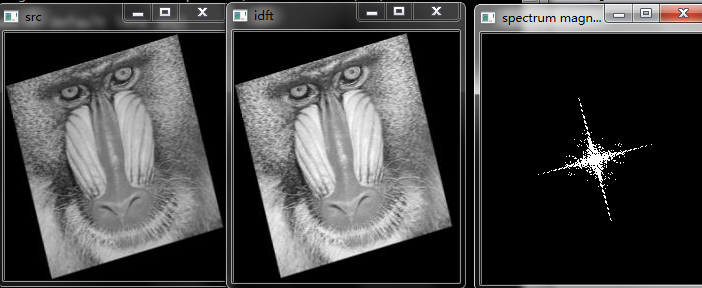展开全文• 5.2 矩阵变换 cv::dct(InputArraysrc,OutputArraydst, int flags=0) 执行一维或二维数组的正向或反向离散余弦变换。 该函数通过查看输入数组的标志大小来选择操作模式: （1）如果(flags & DCT_INVERSE) ==...
5.2 矩阵变换

cv::dct (InputArray src, OutputArray dst, int flags=0)

执行一维或二维数组的正向或反向离散余弦变换。

该函数通过查看输入数组的标志和大小来选择操作模式:

（1）如果(flags & DCT_INVERSE) == 0，则函数执行正向一维或二维转换。否则，它就是一维或二维的逆变换。

（2）如果(flags & DCT_ROWS) != 0，函数对每一行执行一维变换。

（3）如果数组是单列或单行，则函数执行一维变换。

（4）如果以上都不正确，则函数执行2D转换。

cv::dft (InputArray src, OutputArray dst, int flags=0, int nonzeroRows=0)

对一维或二维浮点数组执行正向或反向离散傅里叶变换。

对于真实的(单通道)数据，傅里叶正变换的输出频谱或傅里叶反变换的输入频谱可以用一种称为CCS(复共轭对称)的压缩格式表示。它是从IPL (Intel*图像处理库)借鉴的。2D CCS频谱是这样的: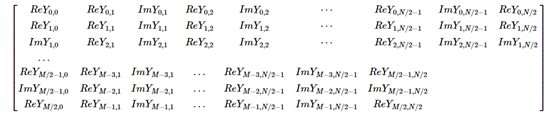cv::divide (InputArray src1, InputArray src2, OutputArray dst, double scale=1, int dtype=-1)

cv::divide (double scale, InputArray src2, OutputArray dst, int dtype=-1)

按数组对两个数组或标量的每个元素进行除法。

cv::eigen (InputArray src, OutputArray eigenvalues, OutputArray eigenvectors=noArray())

计算对称矩阵的特征值和特征向量。

函数cv::eigen只计算特征值，或者说对称矩阵src的特征值和特征向量:

src*eigenvectors.row(i).t() = eigenvalues.at<srcType>(i)*eigenvectors.row(i).t()

cv::eigenNonSymmetric (InputArray src, OutputArray eigenvalues, OutputArray eigenvectors)

计算非对称矩阵的特征值和特征向量(仅实特征值)。

cv::flip (InputArray src, OutputArray dst, int flipCode)

围绕垂直、水平或两个轴翻转二维数组。

函数cv::flip以三种不同的方式翻转数组(行和列索引都是基于0的)之一: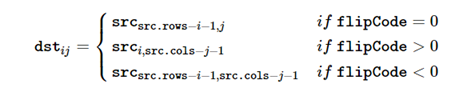使用该功能的示例场景如下：垂直翻转图像（flipCode == 0）以在左上角和左下角图像原点之间切换。这是Microsoft Windows * OS上视频处理的典型操作。使用随后的水平移位和绝对差值计算来水平翻转图像以检查垂直轴对称（flipCode> 0）。同时水平和垂直翻转图像，随后进行移位和绝对差值计算，以检查中心对称性（flipCode <0）。反转点数组的顺序（flipCode> 0或flipCode == 0）。

参数

src

输入数组。

dst

输出与src大小和类型相同的数组。

flipCode

用于指定如何翻转数组的标志; 0表示绕x轴翻转，正值（例如1）表示绕y轴翻转。负值（例如，-1）表示在两个轴周围翻转。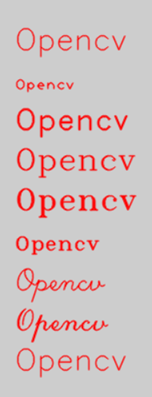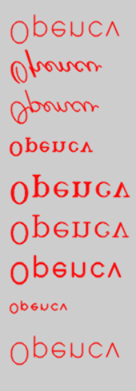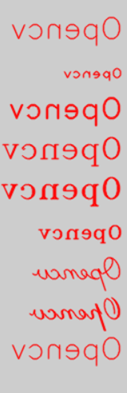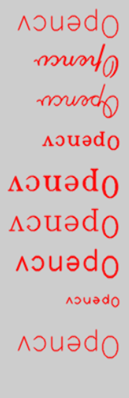cv::idct (InputArray src, OutputArray dst, int flags=0)

计算一维或二维数组的反离散余弦变换。

cv::idft (InputArray src, OutputArray dst, int flags=0, int nonzeroRows=0)

计算一维或二维数组的离散傅里叶反变换。

cv::mulTransposed (InputArray src, OutputArray dst, bool aTa, InputArray delta=noArray(), double scale=1, int dtype=-1)

计算矩阵的乘积及其移位。

cv::perspectiveTransform (InputArray src, OutputArray dst, InputArray m)

执行向量的透视矩阵变换。

cv::transform (InputArray src, OutputArray dst, InputArray m)

执行每个数组元素的矩阵转换。

函数cv::transform对数组src的每个元素进行矩阵变换，并将结果存储在dst中:

m.cols=src.channels()：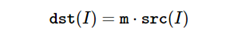m.cols=src.channels()+1：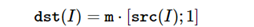N通道阵列src的每个元素都被解释为N元素向量，该N元素向量使用M x N或M x (N+1)矩阵M转换为M元素向量——输出阵列dst的对应元素。

该函数可用于N维点的几何变换、任意线性颜色空间变换(如各种RGB到YUV变换)、图像通道的变换等。

cv::transpose (InputArray src, OutputArray dst)

转置一个矩阵。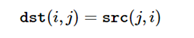cv::vconcat (const Mat *src, size_t nsrc, OutputArray dst)

将垂直连接应用于给定的矩阵。

该函数垂直连接两个或多个cv::Mat矩阵(具有相同数量的cols)。

cv::Mat matArray[] = { cv::Mat(1, 4, CV_8UC1, cv::Scalar(1)),
cv::Mat(1, 4, CV_8UC1, cv::Scalar(2)),
cv::Mat(1, 4, CV_8UC1, cv::Scalar(3)),};
cv::Mat out;
cv::vconcat( matArray, 3, out );
//out:
//[1,   1,   1,   1;
// 2,   2,   2,   2;
// 3,   3,   3,   3]


5.3 其他

cv::borderInterpolate (int p, int len, int borderType)

计算外推像素的源位置。

当使用指定的外推边界模式时，该函数计算并返回与指定外推像素对应的给定像素的坐标。例如，如果您在水平方向使用cv::BORDER_WRAP模式，在垂直方向使用cv::BORDER_REFLECT_101，并且想要计算浮点图像img中的“虚拟”像素点(- 5,100)的值，如下：

float val = img.at<float>(borderInterpolate(100, img.rows, cv::BORDER_REFLECT_101),
borderInterpolate(-5, img.cols, cv::BORDER_WRAP));


cv::cartToPolar(InputArray x,InputArray y,OutputArray magnitude,OutputArray angle,bool angleInDegrees = false）

计算二维向量的大小和角度。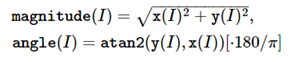参数

x

x坐标数组; 这必须是单精度或双精度浮点数组。

y

y坐标数组，必须与x具有相同的大小和相同的类型。

magnitude

输出与x相同大小和类型的数量的数组。

angle

输出与x具有相同大小和类型的角度数组; 角度以弧度（从0到2 * Pi）或以度（0到360度）测量。

angleInDegrees

一个标志，指示角度是以弧度（默认情况下）或以度为单位测量的。

cv::copyMakeBorder (InputArray src, OutputArray dst, int top, int bottom, int left, int right, int borderType, const Scalar &value=Scalar())

在图像周围形成边框。

该函数将源图像复制到目标图像的中间。复制的源图像的左边、右边、上面和下面的区域将被外推像素填充。这不是基于它的过滤函数所做的(它们动态地推断像素)，而是其他更复杂的函数，包括您自己的函数，可能会做什么来简化图像边界处理。

当src已经位于dst中间时，该函数支持该模式。在这种情况下，函数并不复制src本身，而是简单地构造边界，例如:

// 让边框在所有方向都相同
int border=2;
//构造一个更大的图像以适合图像和边框
Mat gray_buf(rgb.rows + border*2, rgb.cols + border*2, rgb.depth());
// 选择它的中间部分没有复制数据
Mat gray(gray_canvas, Rect(border, border, rgb.cols, rgb.rows));
//将图像从RGB转换为灰度
cvtColor(rgb, gray, COLOR_RGB2GRAY);
// 在适当的位置形成一个边框
copyMakeBorder(gray, gray_buf, border, border,
border, border, BORDER_REPLICATE);
// 现在做一些自定义过滤…
...


cv::countNonZero (InputArray src)

该函数返回src中非零元素的数量

cv::PSNR (InputArray src1, InputArray src2)

计算峰值信噪比(PSNR)图像质量度量。

该函数计算两个输入阵列src1和src2之间的峰值信噪比(PSNR)图像质量度量，单位为分贝(dB)。数组必须有深度CV_8U。

cv::solve (InputArray src1, InputArray src2, OutputArray dst, int flags=DECOMP_LU)

函数cv::solve解决线性系统或最小二乘问题(后者可以使用SVD或QR方法，或者指定标志DECOMP_NORMAL):

cv::solveCubic (InputArray coeffs, OutputArray roots)

求一个三次方程的实根。

cv::solvePoly (InputArray coeffs, OutputArray roots, int maxIters=300)

求多项式方程的实根或复根。

展开全文OPencv
• 文章目录傅里叶级数与傅里叶变换离散傅里叶变换 ...离散信号周期信号最重要的关联在于它可以用一组有限的数字表示。因此，可以使用数字系统来实现DFT。 这个求和的结果恰好落在实值轴上或虚轴上。 ...
• 利用DFT的向量／矩阵表示式： 　也就是可以利用刻度为1／N的严的DFT计算离散傅立叶反变换。 　1．实序列的DFT 　现在来研究一下当输入序列是实数时，一些DFTFFT）计算的额外简化计算。在这种情况下，我们有...
• FourierTransform2D ... Project利用MPI执行正向反向2D DFT。 程序使用阻塞的发送接收功能在CPU之间复制矩阵块以进行计算。 程序当前从特定格式的文本文件中读取图像。 它还要求行列的数量是CPU数量的倍数。C++
• DFT：编写用于计算正向傅立叶变换，逆傅立叶变换，离散余弦变换傅立叶变换幅度的代码。 程序的输入是大小为15X15的2D矩阵DFT / DFT.py：编辑函数“ forward_transform”，“ inverse_transform”，“ discrete_...
• 因为是三进制不进位加法，所以三进制下每...可以用矩阵树定理，矩阵的每个元素是一个三元组，表示方案数 这样转移的常数是9，然而数据好像只有小于等于40的… 转移实际上是一个循环卷积的形式，考虑DFT 那么就要找到
• 我创建了Python版本的DFT和逆DFT算法，以复习DFT的概念。 dft.py def dft(input) ：用于根据时域阵列input计算频谱S的函数。 根据公式S = V^H * s ，其中S是频谱， V^H是DFT矩阵的厄米（hermitian），并且input s ...
• X V 之间估计的偏移量也用于对齐另一个矩阵 Y。 请运行 demo.m 以查看示例。 它在诸如光学相干断层扫描之类的应用中很有用，在这些应用中，由于激光不稳定性而存在信号（X 列）的扫描到扫描变化。 版本历史： - ...matlab
• DFT的matlab源代码ElasTool是一个基于Python的自动化工具包，用于计算属于二维三维的任何晶体系统的二阶弹性常数（SOEC）。 它利用三种应变矩阵集：高效应变矩阵集（OHESS），通用线性独立耦合应变（ULICS）...
• 0，N维复矢量具有DFT和IDFT，DFT和IDFT又是N维复矢量。 逆变换由下式给出： 查看DFT的另一种方法是注意，在以上讨论中，DFT可以表示为DFT矩阵，即Sylvester在1867年引入的Vandermonde矩阵，DFT矩阵定义为 在哪里 FFT...
• 为解决多速率多载波扩频多址系统存在的信道衰落条件下性能下降以及频谱效率相对较低的问题，利用矩阵置换原理 r 循环信道矩阵的分解特性，提出一种自适应多速率 DFT 加扰矢量多载波码分多址接入（AMR-DFT-VMC-CDMA...正交频分复用
• ## dft转换与反转

千次阅读 2014-03-12 14:58:29
在图像处理领域，通过DFT可以将图像转换到频域，实现高通低通滤波；还可以利用矩阵的卷积运算等同于其在频域的乘法运算从而优化算法降低运算量， 即先将图像转换到频域，然后做完乘法运算后，再转换到图像域，...
• 离散傅里叶变换（DFT）1....对于一组标准正交基标准正交基矩阵，对于任意的信号，我们有以下的表达：综合式：分析式： 或 综合式表明信号可以表示成标准正交基的线性组合。分析式给出了计算标准正...数值分析 机器学习 计算机视觉 线性代数
• DFT的matlab源代码改进的HEAAN 原始的HEAAN在。 该代码用于论文“快速同态离散傅立叶变换改进的FHE自举”（）。 相依性 该库由c ...test_homomorphic_dft“ test_improved_bootstrapping”文件。
• 例2：通过DFT和IDFT处理另一个一维离散信号-cx2.m和cx2_sx.m 例3：对时域信号进行DFT，然后将其转换为频域信号。 然后对频域信号进行滤波-cx3.m和cx3_sx.m 信号的循环卷积-cyclic_convolution.m 二维DFT后的频幅图...
• 傅里叶变换 矩阵 DFT-dftmtx 是对称 Vandermonder Matrix conj(dftmtx )=dftmtx '; IDFT-conj(dftmtx )/N fft(x)-------conj(dftmtx )*x % x column vector ifft(x)--------conj(dftmtx )/N*x % fft ...fft matrix
• ＤＦＴ作了扩展，得到在单位圆内的各同心圆上做Ｚ变换的快速算法，并利用扩展的ＤＦＴ提出了一种快速的Ｐｒｏｎｙ算法，改进了现有Ｐｒｏｎｙ算法存在的计算速度慢、所占内存大、且涉及复矩阵运算的问题。...
• DFT的matlab源代码神经网络 ř浮选我nvariant CNN（RiCNN）是神经网络完成旋转等变卷积全球旋转不变性的类型。...第二个方法获取网络的最终卷积特征图，并应用完全连接的旋转权重矩阵，将输入特征图中的旋转转换为输
• dft函数的作用是对一维或者二维浮点数数组进行正向或反向离散傅里叶变换 void dft(InputArray src,OutputArray dst,int flags =0,int nonzeroRows=0) ...函数调用后的运算结果存在这里，其尺寸类型取决于标识符，opencv
• 矩阵.mat收益矩阵 Merv1.mat数据 MFit3.mat参数适合每个人 第7章程序 文件名：DFT模型 DFT2ALTCRT计算2 alt模型的选择概率时间 PlotDTChoiceTime地块分布S MDFT主要的多种替代方案 fitMDFT适合多种替代DFT simMDF...
• 该程序从格式化的检查点文件（.fchk）中读取规范的分子轨道系数矩阵，并输出具有以下名称CorrespondingOrbitals-filename.fchk的新.fchk文件。 该程序还将打印结果摘要，其中包括轨道号。 占用率alpha-beta重叠。 ......